[Contents]

Cause and Effect
John Denker

## 1  Introduction

First, let me explain why it is important to think clearly about cause-and-effect relationships.

It turns out that judgments about cause-and-effect often lead to real-world actions. For example, suppose you find that certain locations are associated with a high number of weird diseases, and then consider the following contrast:

 If there is something about the location (e.g. Picher, Oklahoma) that is causing weird diseases, then you might well decide to take drastic action, such as moving everybody out. If the location (e.g. the Mayo Clinic) is not causing the diseases, then it would be a really bad idea to move everybody out.

These are not made-up examples: Anybody in ZIP code 74360 (Picher) would be better off almost anywhere else, while anybody in ZIP code 55905 (Mayo Clinic) would be worse off almost anywhere else.

There are innumerable other examples where one must determine whether there is a causal relationship between two things. Large numbers of lives and dollars depend on such determinations every day.

In industry, in government, and in ordinary daily life people need to make judgments about what causes what. Sometimes the stakes are small, but sometimes the stakes are enormous:

• will XYZ vaccine cause a large reduction in disease?
• will putting bomb-detectors in airports cause a reduction in the number of bombings?
• ... and in each case will the reductions be worth the cost?

In a democracy, it is important for more-or-less everybody to be able to think clearly about such issues.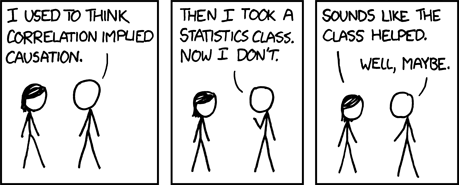Figure 1: Correlation ± Causation [xkcd]

## 2  Causation, Implication, and Related Notions

Why did Jones break his leg?
“Because his tibia hit the kerb,” says the surgeon.
“Because some fool dropped a banana skin on the road,” says Mrs. Jones.
“Because he never looks where he goes,” says a colleague.
“Because he subconsciously wanted a holiday,” says a psychiatrist.
– Otto R. Frisch, in reference 1

The notion of cause-and-effect is more subtle than the notions of ordinary logic, as we now discuss:

The following three ideas are equivalent:

• Plain English: If A then B.
• Formal logic: AB   [pronounced “A implies B”]
• Boolean algebra: B + ∼ A   [pronounced “B or not A”]

Those are closely related to (i.e. a limiting case of):

• Probability: P(B|A)   [pronounced “probability of B given A”]

However, as we shall see, those four ideas are not anywhere close to

• A causes B”.

To see this, consider the following example:

A” means: person developed rabies symptoms
B” means: person was bitten by wild animal

I have a dataset with many rows (one for each person) and two columns (A and B). Within this dataset we observe that AB. You can check that in every row, we either have B or ∼ A; that is, either the person was bitten, or the person did not show rabies symptoms.

On the other hand, it would be absurd to suppose that A causes B. The person’s rabies symptoms are not the cause of the bite. The cause cannot happen after the effect.

We conclude that in general, it is not safe to infer a cause-and-effect relationship from an ordinary collection of data. You can infer that A implies B, but not that A causes B.

Even if you have timing information as well as statistical information

t(dawn) > t(cock-crowing) and
P(dawn | cock-crowing) > 0.9999

you cannot safely infer that the rooster caused the dawn.

Correlation does not imply causation. Implication does not imply causation. It has been understood for thousands of years that «post hoc ergo propter hoc» is a gross fallacy. The pitfalls of not properly making such distinctions are discussed in Feynman’s sermon about cargo-cult science. The most widely-available source for this is reference 2.

## 3  Defining Causation, Inferring Causation

Now that we have seen some of the pitfalls, let us move into positive territory and see what we can properly say about causation.

This is not easy. Experts have struggled for centuries, trying to find an exact definition of causality. The best modern work on this topic has its roots in epidemiology, and borrows some colorful terminology from that field. For instance, the term “intervention” corresponds essentially to what a physicist would call a “perturbation”. Meanwhile the term “counterfactual” corresponds essentially to what a physicist would call “hypothetical”.

For some good modern work in this area, rich in technical details, see reference 3. A nice “data driven” approach can be seen in reference 4, especially reference 5.

To a first approximation, in simple cases we can say the following: A statement about causation is a predictive hypothesis: it says you cannot remove the effect without removing the cause. To say the same thing the other way: given any intervention that allows the cause to remain, the effect will remain.

Here we begin to see the difference between a statement about statistics and a statement about causation.

• Statistics allows you to verify an implication, A implies B, and this naturally leads to the prediction that if you draw more samples from the same distribution, you will continue to observe B or not A in future data.
• A statement about causation makes a much bolder prediction. The statement X causes Y suggests that even if you intervene so as to change the distribution, you will observe either Y or not X in samples drawn from the new distribution.

 In simple cases, any change that removes the effect must have removed the cause.

This applies in simple cases, where there is a single cause and a direct cause-and-effect relationship. More complex cases will be discussed below.

Like most other scientific hypotheses, such statements about causation cannot be proved, only disproved. If a hypothesis is repeatedly tested and not disproved, we gradually raise our estimate of the reliability of the hypothesis. It is possible (under certain assumptions) to quantify the reliability of such hypotheses, but the details are beyond the scope of this discussion.

For example, if we trick the rooster into crowing two hours early (reference 6), we have disproved the hypothesis that cock-crowing causes the dawn.

As a contrasting example, suppose we intervene to reduce the number of people who get bitten, perhaps by teaching people to stay away from wild animals. That will reduce the incidence of human rabies symptoms, OTBE1 (Other Things Being Equal). This is consistent with all of our hypotheses, so it neither proves or disproves anything. Remember, causation is disproved if we can remove the alleged effect without removing the alleged cause, and that didn’t happen in this scenario. Both variables declined together, so nothing is disproved.

Most cause-and-effect relationships involve multiple factors. Sometimes there are factors in series: You get rabies symptoms if you get bitten and the animal was infected and you don’t get treatment. Sometimes there are factors in parallel: you can cause a piece of paper to separate into two pieces by ripping it or cutting it with a knife or cutting it with scissors.

We must be careful when testing causation hypotheses if there are multiple factors involved, as we see from the following scenario: Suppose we intervene by vaccinating a bunch of people, and then take some more data. We find a reduction in the number of people with rabies symptoms, without any reduction the number of people bitten by wild animals. This disproves the hypothesis that getting bitten is the sole causative factor, so we must turn our attention to hypotheses that multiple factors in series.

Things get very tricky if there are are multiple low-probability factors in series, or if there are multiple high-probability factors in parallel.

Note 1: Of course all the rabies hypotheses considered here are oversimplified – but they are not wrong and could be used as the basis of non-ridiculous (albeit imperfect) policy decisions.

Note 2: We can do a pretty good job of evaluating the various hypotheses without knowing anything about the temporal ordering of the bites and the rabies symptoms. On the other hand, if we do have timing information we can use it to bolster the conclusion.

These examples illustrate the general rule that to infer a cause-and-effect relationship, you need to study how the system responds to interventions (i.e. perturbations).

You need to be careful to specify the details of the intervention.

You also need to be careful to specify what OTBE means. As an illustration of this pitfall, consider a lever with four labeled points: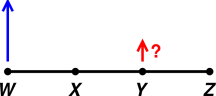Figure 2: Multi-Fulcrum Lever

and we want to know whether point Y rises when I raise point W. Well, that rather depends on whether the fulcrum is at point X or point Z! To repeat: it does not suffice to say that the intervention is raising W. Raising W at constant X is very different from raising W at constant Z.

## 4  Equivalence Relations

Before we go much farther, we ought to say explicitly what we mean by “equality”.

In the fields of mathematics and formal logic, there exists the concept of an equivalence relation. A relation X(⋯,⋯) between two items is an equivalence relation if it is reflexive, symmetric, and transitive:

• Reflexive: X(A,A) is true ... for all A.
• Symmetric: If X(A,B) then X(B,A) ... for all A and B.
• Transitive: If X(A,B) and X(B,C) then X(A,C) ... for all A, B, and C.

The familiar and obvious example of such a relationship is the usual “equality” operator:

• Reflexive: A=A always.
• Symmetric: If A=B then B=A.
• Transitive: If A=B and B=C then A=C.

Note that an equivalence relationship need not – and usually does not – depend on every aspect of the items. For example, if we have a red triangle, a green triangle, a blue triangle, and a blue square, as shown in figure 3, the two triangles are equivalent with respect to number of vertices, while the two blue items are equivalent with respect to color.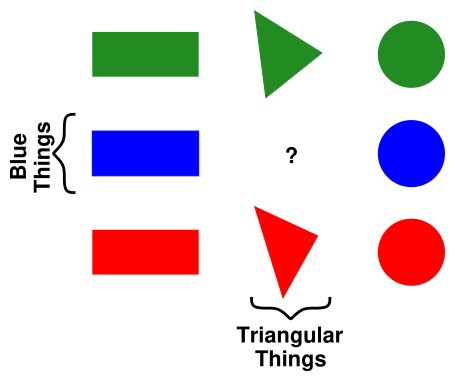Figure 3: Two Different Equivalence Classes

Given an equivalence relationship, we can define the idea of equivalence class. A give set is an equivalence class if every pair of members of the class satisfy a given equivalence relationship. For example, if the equivalence relationship is “same number of vertices”, all triangles are members of one equivalence class while all quadrilaterals are members of a different equivalence class.

As an instructive example of an operator that does not express an equivalence relationship, consider the notion of proximity. It is reflexive (because A is always near A) and symmetric (because if A is near B, B is near A), but it is not transitive. There are plenty of cases where A is near B and B is near C, but A is not near C. This give rise to the proverb:

 Proximity is not transitive.

## 5  Terminology

Beware of the following contrast:

 In non-scientific language and thought, there is a close connection between “force” and “cause”, as we see from the similarity of meaning in the following sentences:“They caused him to do something.”“They forced him to do something.” When it comes to physics, it is a horrible mistake to confuse force with cause. Anything that blurs the distinction between force and cause is a big step in the wrong direction.

 Similar remarks could be made about numerous other words, including “pressured”, “impelled”, et cetera. In particular, as we shall see in section 9, it is a mistake to think that the equation F=ma means F causes ma (or vice versa).

 Given this conflict between homespun terminology and technical terminology, it is unsurprising that non-experts are often confused about the distinction between force and cause.

## 6  Feedback Loops

In some informal sense, every element in a feedback loop is both a cause and an effect. This applies to negative feedback as well as positive feedback. The naïve assumption that causes must be distinct from effects is untenable.

Informally, this does not violate the requirement that cause should precede effect, because if you disturb any given element of the feedback loop, the effects ripple around the loop over time, affecting other elements, eventually affecting the given element itself.

Consider the RS latch shown in figure 4. Normally the R and S inputs are at a “high” logic level. A low-going pulse will force output B high. Normally output A will go low, and the system will latch up in that state. Conversely, a low-going pulse on input S will force output A high. Normally output B will go low, and the system will latch up in that state.

Hint: S stands for “set” and R stands for “reset”.

When the system is latched up, it is impossible to identify (or even define) a “cause” or an “effect” in such a system, in terms of conventional, formal ideas. Testing to see what happens if we remove the cause doesn’t work, since the cause – namely a low-going input pulse – has long since been removed.

(If we get to watch a sufficiently rich history of such a system, we may be able to identify the causative factors.)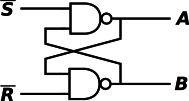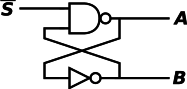Figure 4: RS Latch Figure 5: S Latch

The S-latch shown in figure 5 is even nastier. A low-going pulse on the S input will cause the system to lock up in the state where A is high and B is low. There is simply no way to unlock it.

Here’s another example: Figure 6 shows a simplified version of a feedback loop that shows up in educational psychology. Other feedback loops are very common in technology; for example, the thermostat on a household heating/cooling system is part of a feedback loop. There are also feedback loops involved in climate change, including some negative feedback paths along with some very scary positive feedback paths.

In the figure, red and black are used to show the elements of the feedback loop, and the interactions between them. Gray is used to show external causative factors, external to the loop.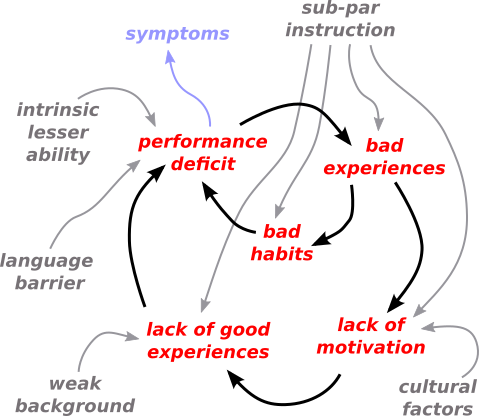Figure 6: Positive Feedback Loop, Plus External Factors

The external causative factors are just causes, not effects. In contrast, each element internal to the loop is both a cause and an effect. Because this is a feedback loop, once it gets going it keeps going, even if you remove all of the external causes. At this point, examining the loop will not tell you what the original external cause(s) might have been. The present situation is controlled by internal causes, internal to the loop.

What’s worse, at this level of detail, figure 6 is just as irreversible as figure 5. Consider the contrast:

 This is a vicious cycle. In other words, it is a self-reinforcing feedback loop. As will all such loops, it is easier to prevent them than to cure them.

 The figure does not show any way to unlatch the feedback loop, once it gets latched up. One hopes that there are other factors, not shown in the diagram, that will allow us to unlatch the loop.

From a policy and planning point of view, you would very much like to know what the original causative factors were, so you can take action to prevent similar feedback loops from starting up in the future. However, you can’t figure that out by looking at the present state of this loop. Furthermore, knowing the original cause(s) doesn’t necessarily tell you how to change the state of this loop as it stands.

Also in figure 6 are symptoms, shown in light blue. These are external effects. They are not causes, and not part of the feedback loop.

Applying some intervention to scrub away the symptom does not change the cause-and-effect relationships within the loop. For example, suppose you find some observable quantity that is initially a 100% reliable symptom, initially 100% correlated with some problem you care about. If you scrub away the symptom, it does not cure the problem. All it does is break the correlation, making the quantity no longer a reliable symptom.

We can deepen our understanding of this with the help of figure 7.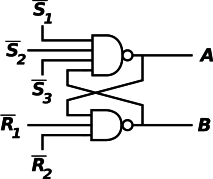Figure 7: RS Latch, Multiple Inputs

In this circuit, there are three different inputs that could set the latch, plus two inputs that could reset the latch. Suppose it is latched in the A state and but we desire to reset it to the B state. We proceed as follows:

1. If any of the causative factors (S1, S2, or S3) is still being applied (S low), then in this case there is a clear cause, explaining the state of the latch. Since we wish to reset the latch, the first order of business is to remove whatever causes are setting it.
2. If/when none of the S inputs is trying to set the latch, the next order of business is to apply a suitable signal to one of the reset inputs (R1 or R2). That is a sufficient cause that will reset the latch.

Normally, when there are no S or R factors acting on the latch, you cannot determine by looking at the present state of the latch which of the inputs “caused” the current situation. If the latch is set, you don’t know which of the three possible inputs set it. If the latch is reset, you don’t know which of the two possible inputs reset it. Either way, the cause is unknowable, lost to history.

• If the only goal was to set or reset the latch, the history is irrelevant. We can dictate the new state without knowing what caused the old state. (This stands contrast to figure 5, where we cannot necessarily dictate the new state.)
• On the other hand, for other purposes – such as policy and planning – it matters a great deal what caused the latch to be set. This is a problem, because the true cause simply cannot be determined by looking at the current state. Sometimes it may be possible to solve the problem by resetting the latch, and then watching it over time, watching closely so that when the latch gets set you know which input caused it.

Much of what was said about the latch circuits applies to the psychological situation in figure 6. In particular, it is entirely possible to have an undesirable self-reinforcing feedback loop that you can get into but cannot get out of. Math anxiety is an example of this (but certaintly not the only example).7 Also, very often it is utterly impossible to ascertain what caused the current state just by looking at the current state.

## 7  The Dawn of Modern Science

It has been known for some time that the basic laws of mechanics do not express causation. The laws of physics must say what happens, but they need not say how it happens, and they almost never say why it happens. This is what sets modern science apart from medieval science. This is what sets physics apart from metaphysics and philosophy. Galileo made a point of this in 1638:

The present does not seem to me to be an opportune time to enter into the investigation of the cause of the acceleration of natural motion, concerning which various philosophers have produced various opinions .... Such fantasies, and others like them, would have to be examined and resolved, with little gain. For the present, it suffices .... to say that in equal times, equal additions of speed are made.
– reference 8, page 203 of the National Edition.

This is tremendously important. In the footnote on page 159 of reference 9, Stillman Drake observes:

Rejection of causal inquiries was Galileo’s most revolutionary proposal in physics, inasmuch as the traditional goal of that science was the determination of causes.

Drake discusses that point at more length in his Introduction (reference 9):

What was lacking in physics, from the time that Aristotle coined that word to name the science of nature, was the idea that actual measurement could contribute anything of real value to any science. The object of science, as set by Aristotle, was to find out the hidden causes of events in nature. Measurement could not reveal underlying causes of the kind required by philosophers, so measurement had no place in physics.

Let me say it again: Galileo’s statement is the epoch, i.e. the defining moment, defining physics as we know it. Modern physics starts from the realization that we don’t need to know whether F causes ma, or vice versa, or both, or neither; for a wide range of purposes it suffices to know that F equals ma.

Newton went to school on Galileo (literally and figuratively). Seventy five years later, Newton expressed the same idea, explicitly disclaiming causation:

Hactenus Phaenomena caelorum et maris nostri per Vim gravitatis exposui, sed causam Gravitatis nondum assignavi.... et Hypotheses non fingo.
Hitherto we have explained the phenomena of the heavens and of our sea by the force of gravity, but have not yet assigned the cause of this force.... and I will not pretend to guess.

There is a time and a place to deal with questions of causation, but introductory mechanics definitely isn’t it. Anything that blurs the distinction between causation and mechanics sets science back almost 400 years.

## 8  Causation in Physics: F=−kx

Let us consider Hooke’s law: F=−kx.

Because equality is symmetric (as discussed in section 4), the equation F=−kx as exactly the same meaning as −kx=F.

There are many ways of applying Hooke’s law. These can be derived from F=−kx using the axiomatic properties of equality, the axiomatic properties of multiplication, and other fundamental mathematical facts.

1. Sometimes we know F and x, and infer k.
2. Sometimes we know x and k, and infer F.
3. Sometimes we know k and F, and infer x.
4. Sometimes we know F, and infer kx without knowing k or x separately.

Item 4 applies to a number of common situations, such a force of constraint. For example, consider a block resting on the table, in equilibrium. We know that the tabletop must be slightly springy, just because all materials are. This allows us to understand the process whereby the block came to be in equilibrium.

We believe that the tabletop has a spring constant k that is very large, but not infinite. We assume that in equilibrium, the block deforms the tabletop by a small amount x, such that the product kx provides just enough force to counterbalance the weight of the block. We do not know the exact value of k or x, and we don’t need to know. The primary requirement is that the product kx must have the right value.

The physics here may be easier to visualize if you place a block on a not-very-taut rubbery drumhead (rather than a tabletop), so that the deformation is large enough to be seen. The magnitude of the deformation can be increased by piling additional weight on the block, and/or by pushing on the block by hand.

Note that when we say a force is a “force of constraint”, that does not make it different from any other force. A force is a force. It does what it does. When we say “of constraint” it is just a hint as to how we expect to calculate the force, probably calculating the force in terms of positions rather than vice versa.

## 9  Causation in Physics: F=ma

### 9.1  F=ma, time reversal, et cetera

The F=ma equation is shorthand for F(t)=ma(t), and means the force at a given time is proportional to the acceleration at the very same time.2 F is a vector, ma is a vector, and the equation states that at any given time, these two vectors are equal. Here equal means the familiar equality relationship discussed in section 4.

With remarkably few exceptions, the laws of physics are invariant under a reversal of the time variable. F(t)=ma(t) has the same meaning as F(−t)=ma(−t).

Exception #1: Thermodynamics has an arrow of time. Some of the laws of thermodynamics are inequalities, not equalities.
Exception #2: Kaon decay is not invariant under time-reversal.
Exception #3: The expansion of the universe means the future is different from the past.
Non-Exception: The causality restrictions of special relativity are invariant under time reversal. The future light cone has the same physics as the past light cone.
Non-Exception: Basic black-hole dynamics is invariant under time-reversal (although there is black-hole thermodynamics also).
Question: Can you think of any other exceptions?

Item #1 (the thermodynamics exception) is the only one I can think of that is even remotely relevant to a first-year mechanics course.

Symmetry arguments contribute a lot to the power (and the elegance) of physics. Lying to students about the time-reversal symmetry of F=ma and the other laws of mechanics is unnecessary and unhelpful, to say the least.

### 9.2  F=ma is not the only thing we know

Sometimes there are scenarios where something involving a force truly causes something involving an acceleration (and not vice versa). But you need to deduce this based on details of the scenario other than F=ma, because there is nothing in the F=ma equation that specifies a direction of causation.

On the other side of the same coin, sometimes there are scenarios where something involving an acceleration truly causes something involving a force (and not vice versa). But you need to deduce this based on details of the scenario other than F=ma.

### 9.3  F=ma plain and simple version

Now, regarding causation itself, various views have been expressed. Here is the plain and simple version. This is the version I recommend.

There is a very simple syllogism:

• Major premise: a cause-and-effect relationship is asymmetric. Causes strictly precede effects, and not vice versa.
• Minor premise: the F=ma equation is symmetric. It has the same meaning as ma=F.
• Conclusion: F=ma does not express a cause-and-effect relationship.
• Stronger conclusion: There cannot be a cause-and-effect relationship between F and ma.

These conclusions are inescapable, given the known laws of motion, and the foregoing major premise.

If a student asks whether F causes ma or vice versa, the answer should be: “You can’t have F without ma, and you can’t have ma without F. The relationship between them is called ’equality’ and is not properly called ’causality’. Equality is symmetric; causality is asymmetric.”

### 9.4  F=ma Big-Endian vs. Little-Endian holy war

Causes strictly precede effects (same as section 9.3).

Big-Endians adjoin to the laws of motion an arbitrary assertion that accelerations are caused by forces.

Little-Endians adjoin to the laws of motion an arbitrary assertion that forces are caused by accelerations.

Each of these is blatantly inconsistent with the known laws of motion, starting with F(t)=ma(t).

(See reference 10 for more about the original holy war between the Big-Endians and the Little-Endians.)

### 9.5  F=ma: Fortran version

Heretofore, the “=” sign signified mathematical equality (an equivalence relation, as discussed in section 4)

However, in this version we let it signify an assignment operator. That is, it represents the relationship “calculated from” rather than “equal to” or “caused by”. This is not an equivalence relation.

In this version, it is easy to come up with examples where F should be calculated from ma, and it is also easy to come up with examples where a should be calculated from F/m. Here are two examples that show the contrast:

 Example: F calculated from ma Example: a calculated from F/m

 Suppose we have a turntable (aka merry-go-round) rotating a a fixed rate. We mark a particular point P on the turntable, fixed relative to the turntable. There is a puck sitting at point P. We can calculate the acceleration a of this point, based on the rotational properties of the turntable. We can then multiply by the mass m of the puck and predict the force F necessary to hold the puck in place. This is an example where F is quite naturally calculated from ma. Suppose we have a rocket of known mass being propelled by a rocket-motor of known thrust. Then we calculate the acceleration from the mass and the force.

 In this example, it is also possible to work backwards, measuring F and m and using that to calculate the acceleration a of the special point P. However, it would be perverse to suggest that F/m was the cause of a (and not vice versa), because you can remove the puck entirely and point P would continue to accelerate just the same.

Just because things are calculated in a given order does not mean that they happen in that order. In fact, F always happens at exactly the same time as ma, no matter how you choose to calculate things.

The F=ma equation cannot shed any light on causality relationships.

Some other computer languages have the advantage of using a less symmetric-looking symbol. They write F := m*a to signify that F is calculated from m times a. This is a conceptual advantage, and you should always think about the “calculated-from” operator as being not symmetric, even if/when it is written using a symmetric-looking symbol.

### 9.6  Cause of Knowing (Not Cause of Happening)

In many cases when somebody asks “why does X happen” the question is open to two interpretations: it could truly be asking for the cause of X ... or it could be asking how we know X will happen.

To repeat: In many cases (but not all), the question “why X” should be deflected. It should be transmuted into something more like:

• “How do we know X?”
• “How could we have predicted X?”
• “What else do we know that is related to X?”

Here’s an example of a sketchy but more-or-less reasonable statement about causation: “I know Jefferson’s inauguration happened in 1801, because the Louisiana Purchase was signed in 1803”. The point here is that if you diagram the sentence, the “because” applies to the knowing, not to the happening. The Louisiana Purchase did not cause the inauguration! It does not explain the inauguration ... but it does explain how I know the date.

Feynman made the point that a chain of deductions need not be ordered the way a chain of causation is; see reference 11.

## 10  Summary

There are at least four ideas in play here:

 symmetric : Equality not symmetric : Causation not symmetric : Defining one thing in terms of another not symmetric : Calculating one thing in terms of another
(1)

Each of these ideas is important, but they are definitely not the same idea.

## 11  References

Many of the online papers mentioned below are in PostScript format. You might find Ghostscript and Ghostview very useful for viewing such documents. The programs are available for free from: http://pages.cs.wisc.edu/ ghost/

1.
Ronald Duncan and Miranda Weston-Smith,
The Encyclopaedia of ignorance : everything you ever wanted to know about the unknown
Pergamon Press (1977)
2.
Richard Feynman,
The Pleasure of Finding Things Out
especially the chapter Cargo Cult Science.
3.
Judea Pearl,
index of papers
http://bayes.cs.ucla.edu/csl_papers.html
4.
David Heckerman,
index of papers
http://research.microsoft.com/en-us/um/people/heckerman/
5.
Heckerman and Shachter,
“Decision-Theoretic Foundations for Causal Reasoning”
http://arxiv.org/pdf/cs.AI/9512104.pdf
6.
Tsuyoshi Shimmura and Takashi Yoshimura,
“Circadian clock determines the timing of rooster crowing”
http://www.sciencedirect.com/science/article/pii/S0960982213001863
7.
Sheila Tobias, Overcoming Math Anxiety W.W. Norton (2nd ed. 1995)
8.
Galileo Galilei,
Discorsi e Dimostrazioni Matematiche intorno à due nuoue scienze Attenenti alla Mecanica & i Movimenti Locali (1638).
9.
Stillman Drake,
Two New Sciences 2nd edition (1989).
(An excellent annotated English translation of reference 8.)
10.
Jonathan Swift,
Gulliver’s Travels
Chapter 5 includes the holy war between the big-endians and the little-endians.
http://www.online-literature.com/swift/gulliver/5/
11.
Richard Feynman,
The Character of Physical Law
especially the passage starting on page 46.

1
Beware that the whole concept of OTBE is fallacious, as discussed in conjunction with figure 2.
2
For simplicity, we assume that the mass is not changing. This assumption works well under a wide range of everyday conditions.
[Contents]